# SET_GSE_ALGEBRAIC_EQN

The SET_GSE_ ALGEBRAIC_EQN subroutine is used with GSESUB to specify the implicit, algebraic equation.

## Use

Setup utility subroutine for GSE.

## Format

Fortran Calling Syntax
SET_GSE_ALGEBRAIC_EQN (gse_id, eqn_idx, errflag)
C/C++ Calling Syntax
c_set_gse_algebraic_eqn (gse_id, eqn_idx, errflag);
Python Calling Syntax
py_set_gse_algebraic_eqn(gse_id, eqn_idx, errflag)

## Attributes

gse_id
[integer]
The identifier of the GSE element.
eqn_idx
[integer]
The equation index of the algebraic equations.

## Output

errflag
[integer]
Returns information about the call status.
A non-zero value indicates failure.

1. All indices are 1-based. That is, the index starts from 1, not 0.
2. SET_GSE_ALGEBRAIC_EQN should only be called when iflag=1.
3. In most cases, GSE are in the following form:
(1)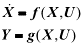However, in some cases, the state equations may involve both differential and algebraic equations. For example:(2)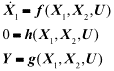When this occurs, it is necessary to tell MotionSolve the equation indices of the algebraic equations associated with the algebraic state X2, using SET_GSE_ ALGEBRAIC_EQN. For example, call: SET_GSE_ALGEBRAIC_EQN (gse_id, 2, errflag)

4. A common use case for SET_GSE_ ALGEBRAIC_EQN is to convert the implicit form of state equations:
(3)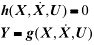into the explicit form that can be solved in MotionSolve. For example, define:(4)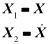The implicit form can be converted to the following differential algebraic equations:(5)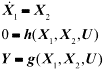This is in the same DAE form shown in the previous remark.